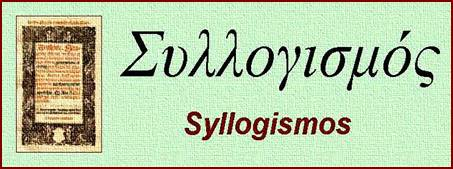History and Hermeneutics for Mathematics Education

Storia ed Ermeneutica per la Didattica della Matematica

A treatise by Carmichael (1855)

Un trattato di Carmichael (1855)Carmichael, R. (1855), A Treatise on the Calculus of Operations, Longman, Brown, Green and Longmans, London

Contents

Chapter I.       Introduction (pp. 1-7)

Chapter II.     Elementary Principles (pp. 8-13)

Chapter III.    Application to the Integration of Linear Total Differential Equations

Section I. Preliminary Theorems (pp. 14-20)

Section II. Application of preceding Theorems (pp. 21-28)

Chapter IV.    Application to the Integration of Linear Partial Differential Equations

Section I. Preliminary Theorems (pp. 29-33)

Section II. Application of preceding Theorems (pp. 34-48)

Chapter V.     Integration of various additional Classes of Differential Equations, Total and Partial (pp. 49-66)

Chapter VI.    Section I. Integration of Systems of Simultaneous Differential Equations (pp. 67-87)

Section II. Evaluation and Extension of Definite Integrals (pp. 88-91)

Chapter VII.   Interpretation of Symbols of Operation (pp. 92-102)

Chapter VIII. Application to Analytic Geometry (pp. 103-124)

Chapter IX.    Micellaneous Applications in the Differential and Integral Calculus (pp. 125-136)

Chapter X.     Application to the Calculus of Finite Differences (pp. 137-152)

Appendix A.  On the Calculus of Variations (pp. 153-160)

Appendix B.  On the Quadrature of Surfaces, and the Rectification of Curves (pp. 161-167)

Appendix C.  Additional Applications to Integration (pp. 168-170)

Introduction.

The Calculus of Operations, in the greatest extension of the phrase, may be regarded as that science which treats of bthe combinations of symbols of operation, conformably to certain given laws, and of the relations by which these symbols are connected with the subjects on which they operate.

As the principal object of the present work is to reduce and simplify the labours of the student, and as the great practical utility of the Calculus of Operations, at present, arises from its bearing upon the Differential and Integral Calculus, and the Calculus of Finite Differences, the symbols of operation employed in illustration, and the laws by which they are governed, and those belonging to the branches of analysis named, and are consequently bfamiliar to the reader” (A Treatise on the Calculus of Operations, p. 1).

Elementary principles.

Two symbols F and G are said to be commutative when, u being the subject on which they operate,

FG u = GF u

A symbol F is said to be distributive when, u and v being two distinct subjects,

F (u+v) = F u + F v

A symbol F is said to be iterative, or to follow the law of indices, when

Fm Fn u = Fm+n u = Fn Fm u

It is to be observed that this third formula is not to be regarded as a law of symbolic combination in the same sense as the first, nor as a law of symbolic operation in the same sense as the second. In fact, Fm u is rather to be regarded as a mere abbreviated notation for the result of the operation F performed m times successively upon u,

F F F ... u

than as equivalent to the result of any operation raised to the power m, performed on u.

The laws stated being the principal ones which occour in the practical employment of the Calculus of Operations, we shall for the present confine our attention to them. They may be called, respectively,

I.       the Law of Commutation

II.      the Law of Distribution

III.     the Law of Indices

We may at once observe, that whatever theorem is true for any one symbol which satisfies these laws is true for every symbol which satisfies them.

Now the symbols of numbers satisfy them; indeed all algebraical equations may be considered as having the same subject, unity, and the constants, as denoting sums of operations performed on unity. Again the symbols of differentiation satisfy those laws; for if u be a function of the two independent variables x and y, it is known that

DxDy u = DyDx u

that, if u be a function of x only,

Dx (u+v) = Dx u + Dx v

and that

Dxm Dxn u = Dxm+n u

Hence we deduce the important consequence, that every theorem in Algebra, which depends on those laws, has an analogue in the Differential Calculus” (A Treatise on the Calculus of Operations, pp. 8-9).

See moreover:

Si veda inoltre:L’Hospital, G. de (1716), Analyse des infiniment petits, Papillon, Paris (II ed.).Newton, I. (1740), Le methode des fluxions et des suites infinites, Debure, Paris (I ed.: 1736).Riccati, V. (1752), De usu motus tractorii in constructione Aequationum Differentialium Commentarius, Lelio della Volpe, Bologna.Paulini a S. Josepho (P. Chelucci) (1755), Institutiones analyticæ earumque usus in Geometria, Gessari, Napoli.Euler, L. (1787) Institutiones Calculi Differentialis cum eius usu in Analysi Finitorum ac Doctrina Serierum, I, II, Galeati, Pavia (II ed.; I ed.: 1755).Euler, L. (1796), Introduction a l’Analyse Infinitésimale, I, II, Barrois, Paris (I ed. in French).Brunacci, V. (1804), Corso di Matematica sublime, I, II, Allegrini, Firenze.Lagrange, J.L. (1813), Théorie des fonctions analytiques, Courcier, Paris.Cauchy, A.L. (1836), Vorlesungen uber die Differenzialrechung, Meyer, Braunschweig.Lacroix, S.F. (1837), Traité elementaire du Calcul Différentiel et du Calcul Intégral, Bachelier, Paris (V ed.).De Morgan, A. (1842), The differential and integral Calculus, Baldwin and Cradock, London.Sturm, Ch. (1868), Cours d’Analyse, I, II, Gauthier-Villars, Paris.Carnot, L.N.M. (1881), Réflections sur la métaphisique du Calcul Infinitésimal, Gauthier-Villars, Paris (I ed.: 1797).Laurent, H. (1885-1887-1888), Traité d’Analyse, I, II, III, Gauthier-Villars, Paris.

Syllogismos.it

History and Hermeneutics for Mathematics Education

(Giorgio T. Bagni, Editor)

Back to Syllogismos.itMain Page

Torna a Syllogismos.it